# SSAT Upper Level Math : How to find the equation of a tangent line

## Example Questions

### Example Question #1 : How To Find The Equation Of A Tangent Line

Circle A is centered about the origin and has a radius of 5. What is the equation of the line that is tangent to Circle A at the point (–3,4)?

3x – 4y = –25

3x + 4y = 7

–3x + 4y = 1

3x – 4y = –1

3x – 4y = –25

Explanation:

The line must be perpendicular to the radius at the point (–3,4). The slope of the radius is given by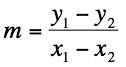The radius has endpoints (–3,4) and the center of the circle (0,0), so its slope is –4/3.

The slope of the tangent line must be perpendicular to the slope of the radius, so the slope of the line is ¾.

The equation of the line is y – 4 = (3/4)(x – (–3))

Rearranging gives us: 3x – 4y = -25

### Example Question #1 : How To Find The Equation Of A Tangent Line

Find the equation of a tangent line at point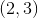if the function is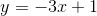.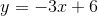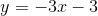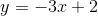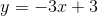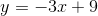Explanation:

To find the slope of the tangent line, it is necessary to determine the slope of the function.

The functionis already in the slope-intercept form,, and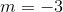.

Substitute the slope and the given pointinto the slope-intercept equation.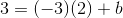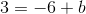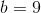Substitute the known slope and the y-intercept to the slope-intercept form.